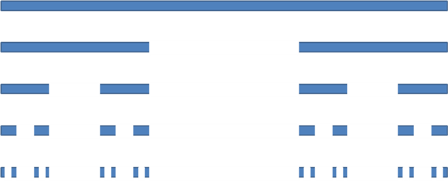The Cantor Set is a fascinating mathematical object.

It is formed by taking the closed interval $[0,1]$ and repeatedly taking the middle third from any line segment.

Therefore at the first iteration the open set $(\frac{1}{3},\frac{2}{3})$ is removed.
 There is a detail to worry about here, as to whether we remove the open set, or the half-open set. Do we remove the left endpoint with the interval, or not. This affects the assertions later about the ternary representation of the points that are in the final set.

At the second iteration two open sets (1/9,2/9) and (7/9,8/9) are removed.

At this point the set consist of the union of four closed intervals namely $[0,\frac{1}{9}]\cup[\frac{2}{9},\frac{3}{9}]\cup[\frac{6}{9},\frac{7}{9}]\cup[\frac{8}{9},1]$

This procedure of taking the open set in the middle of each line segment is repeated ad infinitum. What remains is the Cantor Set.### (Apart from the end points of intervals) Are there any other points in the Cantor Set?

Consider all numbers in the interval [ 0, 1] written in tertiary (base 3).

At the first iteration, numbers with the form 0.1... (base 3) will be removed leaving numbers 0.0...(base 3) and 0.2... (base 3).

At the second iteration numbers with 1 in the second tertiary place will be removed.

At the nth iteration only numbers with 1 in the nth tertiary place will be removed.

Therefore, the Cantor Set consists of all number, in tertiary, with either zero or two in any position after the radix point. e.g. 0.200220222200...

1/4 = 0.0202020202 ... (base 3) is a point in the Cantor Set.

Enrichment

Show that 1/4 is not an end point of intervals.

### What is the size of the Cantor set?

Counting up the length of the interval that are removed at each iteration.

1/3 + 2/9 + 4/27 + 8/81 .... = 1

The "length" removed is 1 however the Cantor set is uncountably infinite.

The Cantor set is fractal.

The analysis and properties of the Cantor set are rich and intersecting.

CountableSet
TransfiniteNumbers
UncountableSet
(none) (none)
GeorgCantor (none)

## You are here

CantorSet
(none)
ContinuumHypothesis
CountableSet
FamousPeople
NaturalNumber
RealNumber
TransfiniteNumbers
UncountableSet
(none) (none)